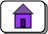Created: 08/07/03

# Basic MIPS Instructions

These are the assembly language statements covered in these notes that each directly correspond to one machine language instruction. There are additional basic assembly language statements that are not covered in these notes.

When pseudoinstructions are enabled, many of these instructions also correspond to pseudoinstructions that have greater flexibility in the arguments that they allow.

In the following, `d, s, ` and `t` are general purpose registers. Register `d` receives the result of operation between the contents of registers `s` and `t` .

InstructionOperandsDescription
```d ← s+t ; with overflow trap
```
```d ← s+t ; without overflow trap
```
```d ← s+const ; with overflow trap
const is 16-bit two's comp
```
```d ← s+const ; without overflow trap
const is 16-bit two's comp
```
and d,s,t
```d ← bitwise AND of s with t
```
andi d,s,const
```d ← bitwise AND of s with const
```
```branch if s == t
A branch delay slot follows the instruction.
```
```Branch if the two's comp. integer
in register s is >= 0
A branch delay slot follows the instruction.
```
```Branch if the two's comp. integer
in register s is < 0
A branch delay slot follows the instruction.
```
```branch if s != t
A branch delay slot follows the instruction.
```
div s,t
```lo ← s div t ; hi ← s mod t
two's comp. operands
```
divu s,t
```lo ← s div t ; hi ← s mod t
unsigned operands
```
j target
```after a delay of one machine cycle,
```
lb d,off(b)
```d ← Sign-extended byte from
off is 16-bit two's complement
```
lbu d,off(b)
```d ← Zero-extended byte
off is 16-bit two's complement
```
lh d,off(b)
```t ← Sign-extended halfword
off is 16-bit two's complement
```
lhu d,off(b)
```t ← Zero-extended halfword
off is 16-bit two's complement
```
lui d,const
```upper two bytes of \$t ← two byte const
lower two bytes of \$t ← 0x0000
```
lw d,off(b)
```d ← Word from memory address b+off
off is 16-bit two's complement.
```
mfhi d
```d ← hi ;  Move From Hi
```
mflo d
```d ← lo ;  Move From Lo
```
mult s,t
```hi | lo ← s * t
two's comp operands
64-bit result in 64-bit pair hi and lo
```
multu s,t
```hi | lo ← s * t
unsigned operands
64-bit result in 64-bit pair hi and lo
```
nor d,s,\$0
```d ← bitwise NOT of s
```
nor d,s,t
```d ← bitwise NOR of s with t
```
or d,s,\$0
```d ← s
```
or d,s,t
```d ← bitwise OR of s with t
```
ori d,\$0,const
```d ← zero-extended const
```
ori d,s,const
```d ← s OR zero-extended const
```
sb d,off(b)
```byte at off+b ← low-order byte from register \$d.
off is 16-bit two's complement
```
sh d,off(b)
```two bytes at off+b ← two low-order bytes
from register \$d.
off is 16-bit two's complement
```
sll \$0,\$0,0
```no operation
```
sll d,s,shft
```d ← logical left shift of s by shft positions
where  0 <= shft < 32
```
slt d,s,t
```if s < t
d ← 1
else
d ← 0

two's comp. operands
```
slti d,s,imm
```if s < imm
d ← 1
else
d ← 0

two's comp. operands
```
sltiu d,s,imm
```if s < imm
d ← 1
else
d ← 0

unsigned operands
```
sltu d,s,t
```if s < t
d ← 1
else
d ← 0

unsigned operands
```
sra d,s,shft
```d ← arithmetic right shift of s by shft positions
where  0 <= shft < 32
```
srl d,s,shft
```d ← logical right shift of s by shft positions
where  0 <= shft < 32
```
sub d,s,t
```d ← s - t; with overflow trap
```
subu d,s,t
```d ← s - t; no overflow trap
```
sw d,off(b)
```Word at memory address (b+off) ← \$t
b is a register. off is 16-bit twos complement.
```
xor d,s,t
```d ← bitwise exclusive or of s with t
```
xori d,s,const
```d ← bitwise exclusive or of s with const
```Return to Home